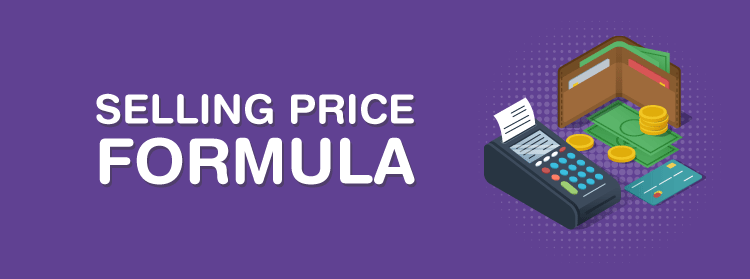# Selling Price Formula

Every day we note a variety of situations where we need to calculate or compare things. in “percent”. Mostly the situations related to selling or buying of items. We use Selling price to sell the article on a particular cost and that can be calculated using the selling price formula.

The amount that a customer pays to buy a product is called a selling Price.## Formula for Selling Price

It can be calculated as follows:

 SP = {(100 + Gain %)/100} x CP SP = {(100 – Loss %)/100} x CP SP = CP + Profit SP = CP – loss
• C.P – Cost Price
• S.P – Selling Price
• If S.P> C.P = Gain
• If S.P < C.P =Loss

Note: The Profit and loss percentage is another important fact to be known for calculating the S.P

## Example Problem Using the Formula of Selling Price

Problem: A seller sells a washing machine at a cost price of Rs 15000 with a profit of 20%. Calculate the price at which the customer will purchase it. And also, find profit earned by the shopkeeper.

Solution:

C.P = Rs 15000

Profit % = 20%

Profit = C.P x profit %

Profit = 15000 X 20/100

Profit = Rs 3,000

Selling price = Profit + C.P

= Rs 3000 + Rs 15,000 = Rs 18,000

So, the amount payable by the customer to the seller = Rs 18,000

Check out more mathematical formulas with practice examples on BYJU’S.

#### 1 Comment

1. Anantharajan

A shopkeeper sells a refrigerators for rs 22,000 and makes a profit of 10%. If he desires to make a profit of 18% what should be his selling price.